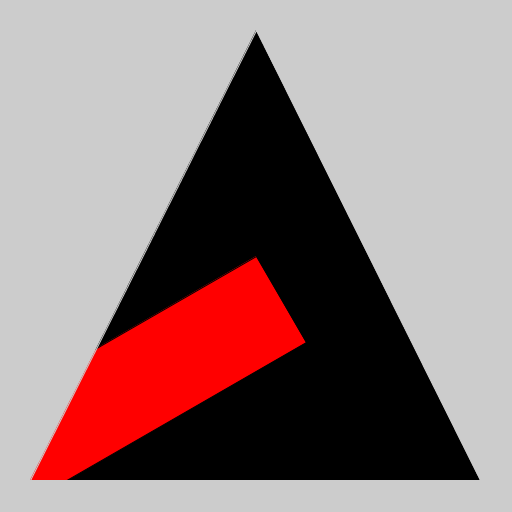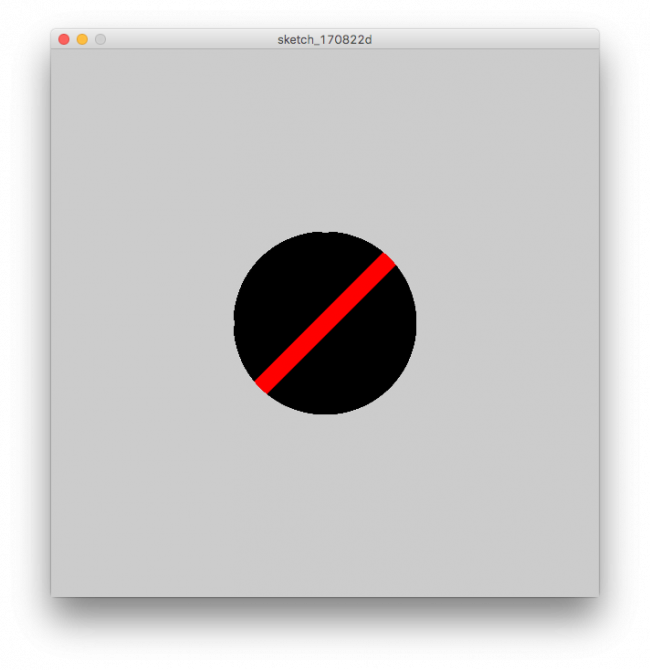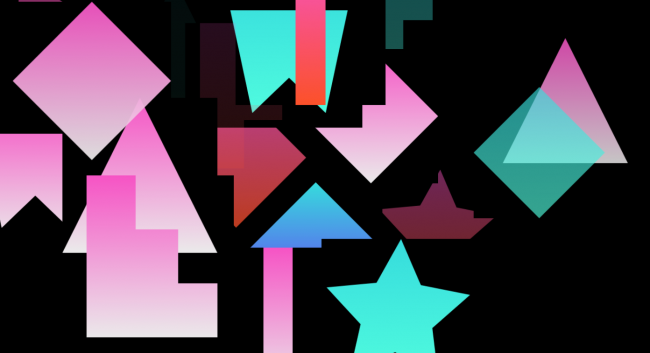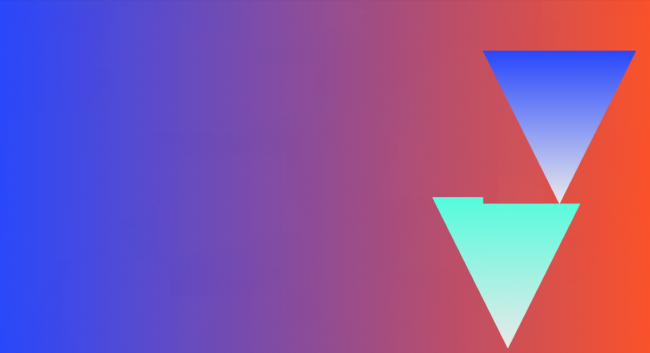#### Howdy, Stranger!

We are about to switch to a new forum software. Until then we have removed the registration on this forum.

# Masking a shape with another shape

edited August 2017

Is it possible to make something like this (below), where the circular shape masks a rectangular shape? I tried this earlier today but I'm having trouble controlling it myself and wondering if there's a simpler way?In the end I'm hoping to make a grid of shapes like that where the colors, width of rectangles, and number of rectangles varies. Thanks in advance for your help.

Tagged:

• @Petros -- yes, it is possible.

Here is an example using an image masked by a triangle:

https://forum.processing.org/two/discussion/18819/how-to-copy-a-triangle-out-of-an-image

But you could instead use a rectangle and mask it with a circle. Same principle.

• Thanks for the tip. I had seen that post but (I'm a total newbie to processing and definitely a non-programmer), couldn't figure out how to switch the photo to something like an orange rectangle (I guess?) for the background. I tried changing the photo variable (which I'm calling 'backing') to a rectangle, but I'm getting an error: "cannot convert from void to PImage". How do I use a color instead of a photo for the background of the masked object?

``````/**
* 2016-11-01 Processing 3.2.1
**/
PImage backing;

void setup() {
size(400, 400);
backing = rect(0,0,400,400);
fill(#F5A623);
}
void draw() {
noLoop();
image(backing, 100, 100);
}
``````
• edited August 2017 Answer ✓

The original example shows loading a PImage photo layer, drawing a shape on a PGraphics mask layer, then combining.

Instead, draw something on a PGraphics image layer, then draw a shape on a PGraphics mask layer, then combine:

``````/**
* Draw a Mask example Pt.2
* 2017-08-22 Processing 3.3.5
**/
PGraphics sourceImage;
void setup() {
size(512, 512);

// create source
sourceImage = createGraphics(512,512);
sourceImage.beginDraw();
sourceImage.fill(255,0,0);
sourceImage.translate(width/2,height/2);
sourceImage.rotate(PI/3);
sourceImage.rect(0,0,100,500);
sourceImage.endDraw();

maskImage.triangle(30, 480, 256, 30, 480, 480);

}
void draw() {
image(sourceImage, 0, 0);
}
``````• Yessss! That is killer thank you! I'm still trying to figure out how to keep the rectangle's position relative to the circle mask; I'll probably try dist() or something like that. Here it is modified a bit to use a circle in case anyone stumbles on this in the future:

``````/**
* Draw a Mask example Pt.2
* 2017-08-22 Processing 3.3.5
**/
PGraphics sourceImage;
void setup() {
size(600, 600); //size of canvas

// create source
sourceImage = createGraphics(600,600); //size of drawing
sourceImage.beginDraw();
sourceImage.fill(255,0,0);
sourceImage.translate(width/2,height/2);
sourceImage.rect(-10,-150,20,500);
sourceImage.endDraw();

}
void draw() {
image(sourceImage, 0, 0);
}
``````• forking this issue. i have created a PGraphics mask and another Pgraphics canvas and passed the masked result to a Surface Object. i also created antoher PGraphics to use as background. I find some issue that's a bit disturbing about the mask. sometimes it works, most of the time, some times it doesn't. when the new Surface is spawned above a pre-existing surface, sometimes it doesn't spawn with the programmed transparency.

here's an image without background. it has some of these flaws.here's an image with canvas as backgroundi've read somewhere, don't rember where about rendering issues with this kind of transparency. can anyone enlight me on this issue?

tks

• Can you share the code for the two triangle demo sketch please? I want to understand exactly what you are doing. First guess it looks like perhaps the masked second triangle is drawn into the surface with blendMode=REPLACE, which would set the pixels of the combined surface to the alpha portion of mask 2.

https://processing.org/reference/blendMode_.html

• it looks like the supposedly transparent background is overwriting the non-transparent parts of the things behind it

i think there's a hint for this, try the following

``````hint(ENABLE_DEPTH_TEST);
``````

or

``````hint(ENABLE_DEPTH_SORT);
``````
• @jeremydouglas i the specific case have, one PGraphic for Background: PGraphics bgG two arrays: PGraphics[] canvas, PGraphics[] mask three functions: void buildGradient, void buildMask and spawnSurface and one Surface Object that moves the masked PGraphics around

I'm initialing the arrays in their own function in setup and i'm not using any blendMode, other than default.

``````ArrayList<Surface> surfaces;

PGraphics bgG;
PGraphics[] canvas=new PGraphics;

void settings() {
size(1392, 756, P3D);
smooth(3);
}

void setup() {
//PGraphics
initBackground();
initCanvas();

surfaces=new ArrayList<Surface>();
}

void draw() {
// background(0);
image(bgG, 0, 0);

for (int i=surfaces.size ()-1; i>=0; i--) {
Surface s=surfaces.get(i);
s.move(pxS, pyS, pzS, axS, ayS, azS);
s.display();
surfaces.remove(i);
}
}
if (isSurface) {
if (frameCount % sSpawn==0) { //sSpawn is a variable that i control using a ControlP5 slider
spawnSurface();
}
}
}

void spawnSurface() {
PVector pos=new PVector(random(width), random(height), random(-100, 100));
PGraphics b=canvas[int(random(canvas.length))];
}

void initBackground() {
bgG=createGraphics(width, height);
buildBackground();
}

void initCanvas() {
for (int i=0; i<canvas.length; i++) {
canvas[i]=createGraphics(mW, mH);
buildCanvas(canvas[i], YAXIS);
}
}

for (int i=0; i<mask.length; i++) {
}
}

void buildBackground() {
buildCanvas(bgG, XAXIS);
bgI=bgG.get();
}

void buildCanvas(PGraphics canvas, int axis) {
canvas.beginDraw();
canvas.background(0,0);
buildGradient(canvas, 0, 0, canvas.width, canvas.height, axis);
canvas.endDraw();
}

void buildCanvas(PGraphics canvas, int axis) {
canvas.beginDraw();
canvas.background(0,0);
buildGradient(canvas, 0, 0, canvas.width, canvas.height, axis);
canvas.endDraw();
}

void buildGradient(PGraphics canvas, int y, int x, int w, int h, int axis) {
color c1=c[int(random(c.length))];
color c2=c[int(random(c.length))];
if (c2==c1) {
c2=c[int(random(c.length))];
}

if (axis == YAXIS) {
for (int i = y; i <= y+h; i++) {
float inter = map(i, y, y+h, 0, 1);
color c3 = lerpColor(c1, c2, inter);
canvas.stroke(c3);
canvas.line(x, i, x+w, i);
}
}
}

switch (mode) {
case 0://arco
mask.arc(w/2, h/2, w, h, -HALF_PI, HALF_PI);
break;
case 1://bola
case 2://diamond
break;
case 3://TRIANGULO
break;
}
``````

this is the Surface object

``````class Surface {
PGraphics g;
PVector p;
int life;
int lifeSpeed;
int imgW, imgH;
float aX=0;
float aY=0;
float aZ=0;

Surface(PVector _pos, PGraphics _g) {
p=_pos.get();
g=_g;
life=500;
lifeSpeed=5;
imgW=g.width/2;
imgH=g.height/2;
}

void run() {
// move();
//display();
}

void move(float pxS, float pyS, float pzS, float axS, float ayS, float azS) {
life-=lifeSpeed;
//all varibles below i
p.x+=pxS;
p.y+=pyS;
p.z+=pzS;
aX+=axS;
aY+=ayS;
aZ+=azS;
}

void display() {
pushMatrix();
translate(p.x, p.y, p.z);
tint(255, life);
image(g, -imgW, -imgH);
popMatrix();
}

if (life<0 || p.x<0 || p.x > width) {
return true;
} else {
return false;
}
}
}
``````
• @koogs

`hint(ENABLE_DEPTH_SORT);`

works perfectly

• Don't know if that's documented anywhere but i think it's forcing a sort of all the objects in the scene and drawing the furthest away first - painters' algorithm... It comes with a performance hit but it shouldn't be much.

• in this case it works perfectly. i used it in another sketch that's more complex and this got heavy.

• If you do all of your compositing in PGraphics then I believe that you don't need to use the hint because it supports alpha -- not sure if that will fix your performance issue, but worth trying.

• @eduzal, I tried to run your code above but it seems to be missing something. Thanks anyway, I kind of get the idea by reading your code where to go next.